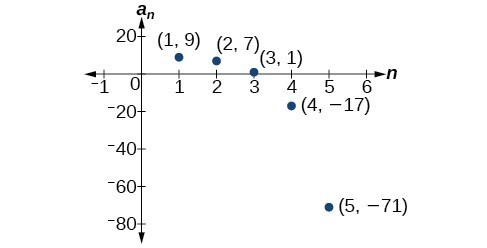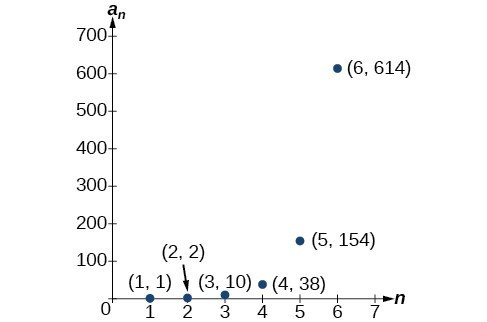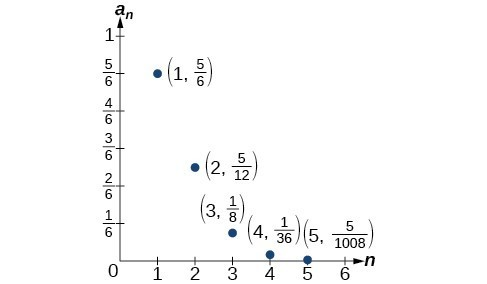## Writing the Terms of a Sequence Defined by a Recursive Formula

Sequences occur naturally in the growth patterns of nautilus shells, pinecones, tree branches, and many other natural structures. We may see the sequence in the leaf or branch arrangement, the number of petals of a flower, or the pattern of the chambers in a nautilus shell. Their growth follows the Fibonacci sequence, a famous sequence in which each term can be found by adding the preceding two terms. The numbers in the sequence are 1, 1, 2, 3, 5, 8, 13, 21, 34,…. Other examples from the natural world that exhibit the Fibonacci sequence are the Calla Lily, which has just one petal, the Black-Eyed Susan with 13 petals, and different varieties of daisies that may have 21 or 34 petals.

Each term of the Fibonacci sequence depends on the terms that come before it. The Fibonacci sequence cannot easily be written using an explicit formula. Instead, we describe the sequence using a recursive formula, a formula that defines the terms of a sequence using previous terms.

A recursive formula always has two parts: the value of an initial term (or terms), and an equation defining ${a}_{n}$ in terms of preceding terms. For example, suppose we know the following:

$\begin{array}{l}{a}_{1}=3\hfill \\ {a}_{n}=2{a}_{n - 1}-1, \text{for} n\ge 2\hfill \end{array}$

We can find the subsequent terms of the sequence using the first term.

$\begin{array}{l}{a}_{1}=3\\ {a}_{2}=2{a}_{1}-1=2\left(3\right)-1=5\\ {a}_{3}=2{a}_{2}-1=2\left(5\right)-1=9\\ {a}_{4}=2{a}_{3}-1=2\left(9\right)-1=17\end{array}$

So the first four terms of the sequence are $\left\{3,\text{ }5,\text{ }9,\text{ }17\right\}$ .

The recursive formula for the Fibonacci sequence states the first two terms and defines each successive term as the sum of the preceding two terms.

$\begin{array}{l}{a}_{1}=1\hfill \\ {a}_{2}=1\hfill \\ {a}_{n}={a}_{n - 1}+{a}_{n - 2}, \text{for} n\ge 3\hfill \end{array}$

To find the tenth term of the sequence, for example, we would need to add the eighth and ninth terms. We were told previously that the eighth and ninth terms are 21 and 34, so

${a}_{10}={a}_{9}+{a}_{8}=34+21=55$

### A General Note: Recursive Formula

A recursive formula is a formula that defines each term of a sequence using preceding term(s). Recursive formulas must always state the initial term, or terms, of the sequence.

### Must the first two terms always be given in a recursive formula?

No. The Fibonacci sequence defines each term using the two preceding terms, but many recursive formulas define each term using only one preceding term. These sequences need only the first term to be defined.

### How To: Given a recursive formula with only the first term provided, write the first $n$ terms of a sequence.

1. Identify the initial term, ${a}_{1}$, which is given as part of the formula. This is the first term.
2. To find the second term, ${a}_{2}$, substitute the initial term into the formula for ${a}_{n - 1}$. Solve.
3. To find the third term, ${a}_{3}$, substitute the second term into the formula. Solve.
4. Repeat until you have solved for the $n\text{th}$ term.

### Example 5: Writing the Terms of a Sequence Defined by a Recursive Formula

Write the first five terms of the sequence defined by the recursive formula.

$\begin{array}{l}\begin{array}{l}\\ {a}_{1}=9\end{array}\hfill \\ {a}_{n}=3{a}_{n - 1}-20\text{, for }n\ge 2\hfill \end{array}$

### Solution

The first term is given in the formula. For each subsequent term, we replace ${a}_{n - 1}$ with the value of the preceding term.

$\begin{array}{ll}n=1\begin{array}{lllll}\hfill & \hfill & \hfill & \hfill & \hfill \end{array}\hfill & {a}_{1}=9\hfill \\ n=2\hfill & {a}_{2}=3{a}_{1}-20=3\left(9\right)-20=27 - 20=7\hfill \\ n=3\hfill & {a}_{3}=3{a}_{2}-20=3\left(7\right)-20=21 - 20=1\hfill \\ n=4\hfill & {a}_{4}=3{a}_{3}-20=3\left(1\right)-20=3 - 20=-17\hfill \\ n=5\hfill & {a}_{5}=3{a}_{4}-20=3\left(-17\right)-20=-51 - 20=-71\hfill \end{array}$

The first five terms are $\left\{9,\text{ }7,\text{ }1,\text{ }-17,\text{ }-71\right\}$.Figure 6

### Try It 7

Write the first five terms of the sequence defined by the recursive formula.

$\begin{array}{l}{a}_{1}=2\\ {a}_{n}=2{a}_{n - 1}+1\text{, for }n\ge 2\end{array}$

### How To: Given a recursive formula with two initial terms, write the first $n$ terms of a sequence.

1. Identify the initial term, ${a}_{1}$, which is given as part of the formula.
2. Identify the second term, ${a}_{2}$, which is given as part of the formula.
3. To find the third term, substitute the initial term and the second term into the formula. Evaluate.
4. Repeat until you have evaluated the $n\text{th}$ term.

### Example 6: Writing the Terms of a Sequence Defined by a Recursive Formula

Write the first six terms of the sequence defined by the recursive formula.

$\begin{array}{l}{a}_{1}=1\\ {a}_{2}=2\\ {a}_{n}=3{a}_{n - 1}+4{a}_{n - 2}\text{, for }n\ge 3\end{array}$

### Solution

The first two terms are given. For each subsequent term, we replace ${a}_{n - 1}$ and ${a}_{n - 2}$ with the values of the two preceding terms.

$\begin{array}{lllllll}n=3\hfill & \hfill & \hfill & \hfill & \hfill & \hfill & {a}_{3}=3{a}_{2}+4{a}_{1}=3\left(2\right)+4\left(1\right)=10\hfill \\ n=4\hfill & \hfill & \hfill & \hfill & \hfill & \hfill & {a}_{4}=3{a}_{3}+4{a}_{2}=3\left(10\right)+4\left(2\right)=38\hfill \\ n=5\hfill & \hfill & \hfill & \hfill & \hfill & \hfill & {a}_{5}=3{a}_{4}+4{a}_{3}=3\left(38\right)+4\left(10\right)=154\hfill \\ n=6\hfill & \hfill & \hfill & \hfill & \hfill & \hfill & {a}_{6}=3{a}_{5}+4{a}_{4}=3\left(154\right)+4\left(38\right)=614\hfill \end{array}$

The first six terms are $\text{{1,2,10,38,154,614}}\text{.}$Figure 7

### Try It 8

Write the first eight terms of the sequence defined by the recursive formula.

$\begin{array}{l}\begin{array}{l}\\ {a}_{1}=0\end{array}\hfill \\ {a}_{2}=1\hfill \\ {a}_{3}=1\hfill \\ {a}_{n}=\frac{{a}_{n - 1}}{{a}_{n - 2}}+{a}_{n - 3}\text{, for }n\ge 4\hfill \end{array}$

## Using Factorial Notation

The formulas for some sequences include products of consecutive positive integers. $n$ factorial, written as $n!$, is the product of the positive integers from 1 to $n$. For example,

$\begin{array}{l}4!=4\cdot 3\cdot 2\cdot 1=24\hfill \\ 5!=5\cdot 4\cdot 3\cdot 2\cdot 1=120\hfill \end{array}$

An example of formula containing a factorial is ${a}_{n}=\left(n+1\right)!$. The sixth term of the sequence can be found by substituting 6 for $n$.

${a}_{6}=\left(6+1\right)!=7!=7\cdot 6\cdot 5\cdot 4\cdot 3\cdot 2\cdot 1=5040$

The factorial of any whole number $n$ is $n\left(n - 1\right)!$ We can therefore also think of $5!$ as $5\cdot 4!\text{.}$

### A General Note: Factorial

n factorial is a mathematical operation that can be defined using a recursive formula. The factorial of $n$, denoted $n!$, is defined for a positive integer $n$ as:

$\begin{array}{l}0!=1\\ 1!=1\\ n!=n\left(n - 1\right)\left(n - 2\right)\cdots \left(2\right)\left(1\right)\text{, for }n\ge 2\end{array}$

The special case $0!$ is defined as $0!=1$.

### Can factorials always be found using a calculator?

No. Factorials get large very quickly—faster than even exponential functions! When the output gets too large for the calculator, it will not be able to calculate the factorial.

### Example 7: Writing the Terms of a Sequence Using Factorials

Write the first five terms of the sequence defined by the explicit formula ${a}_{n}=\frac{5n}{\left(n+2\right)!}$.

### Solution

Substitute $n=1,n=2$, and so on in the formula.

$\begin{array}{lllll}n=1\hfill & \hfill & \hfill & \hfill & {a}_{1}=\frac{5\left(1\right)}{\left(1+2\right)!}=\frac{5}{3!}=\frac{5}{3\cdot 2\cdot 1}=\frac{5}{6}\hfill \\ n=2\hfill & \hfill & \hfill & \hfill & {a}_{2}=\frac{5\left(2\right)}{\left(2+2\right)!}=\frac{10}{4!}=\frac{10}{4\cdot 3\cdot 2\cdot 1}=\frac{5}{12}\hfill \\ n=3\hfill & \hfill & \hfill & \hfill & {a}_{3}=\frac{5\left(3\right)}{\left(3+2\right)!}=\frac{15}{5!}=\frac{15}{5\cdot 4\cdot 3\cdot 2\cdot 1}=\frac{1}{8}\hfill \\ n=4\hfill & \hfill & \hfill & \hfill & {a}_{4}=\frac{5\left(4\right)}{\left(4+2\right)!}=\frac{20}{6!}=\frac{20}{6\cdot 5\cdot 4\cdot 3\cdot 2\cdot 1}=\frac{1}{36}\hfill \\ n=5\hfill & \hfill & \hfill & \hfill & {a}_{5}=\frac{5\left(5\right)}{\left(5+2\right)!}=\frac{25}{7!}=\frac{25}{7\cdot 6\cdot 5\cdot 4\cdot 3\cdot 2\cdot 1}=\frac{5}{1\text{,}008}\hfill \end{array}$

The first five terms are $\left\{\frac{5}{6},\frac{5}{12},\frac{1}{8},\frac{1}{36},\frac{5}{1,008}\right\}$.

### Analysis of the Solution

Figure 8 shows the graph of the sequence. Notice that, since factorials grow very quickly, the presence of the factorial term in the denominator results in the denominator becoming much larger than the numerator as $n$ increases. This means the quotient gets smaller and, as the plot of the terms shows, the terms are decreasing and nearing zero.Figure 8

### Try It 9

Write the first five terms of the sequence defined by the explicit formula ${a}_{n}=\frac{\left(n+1\right)!}{2n}$.

Solution